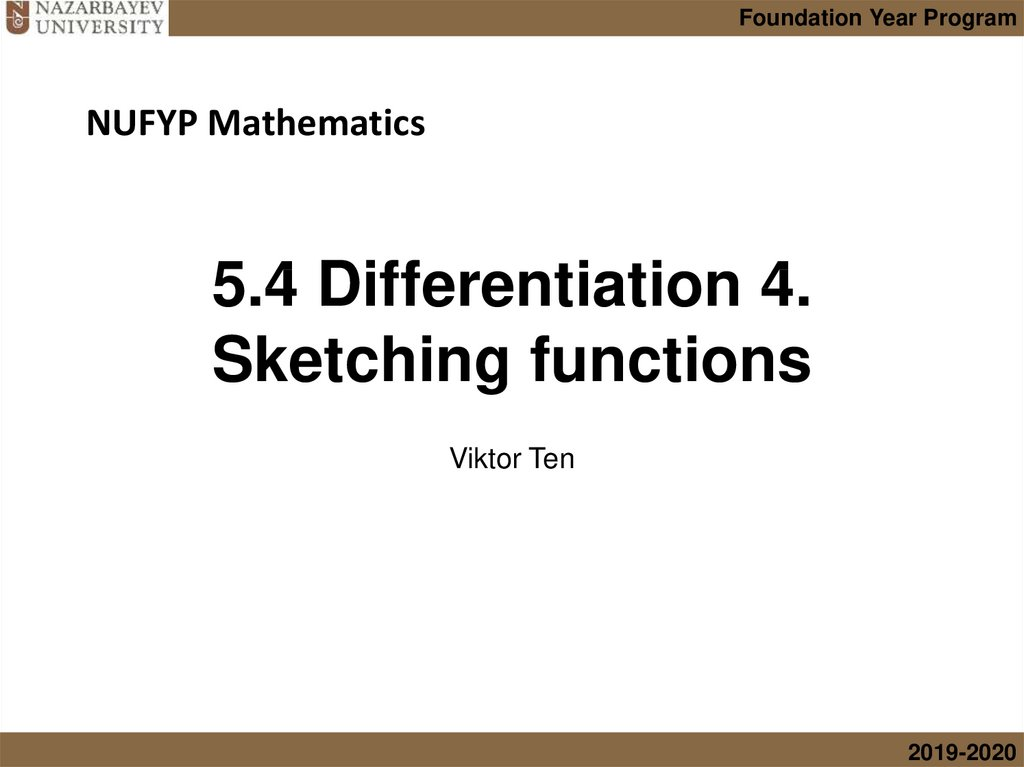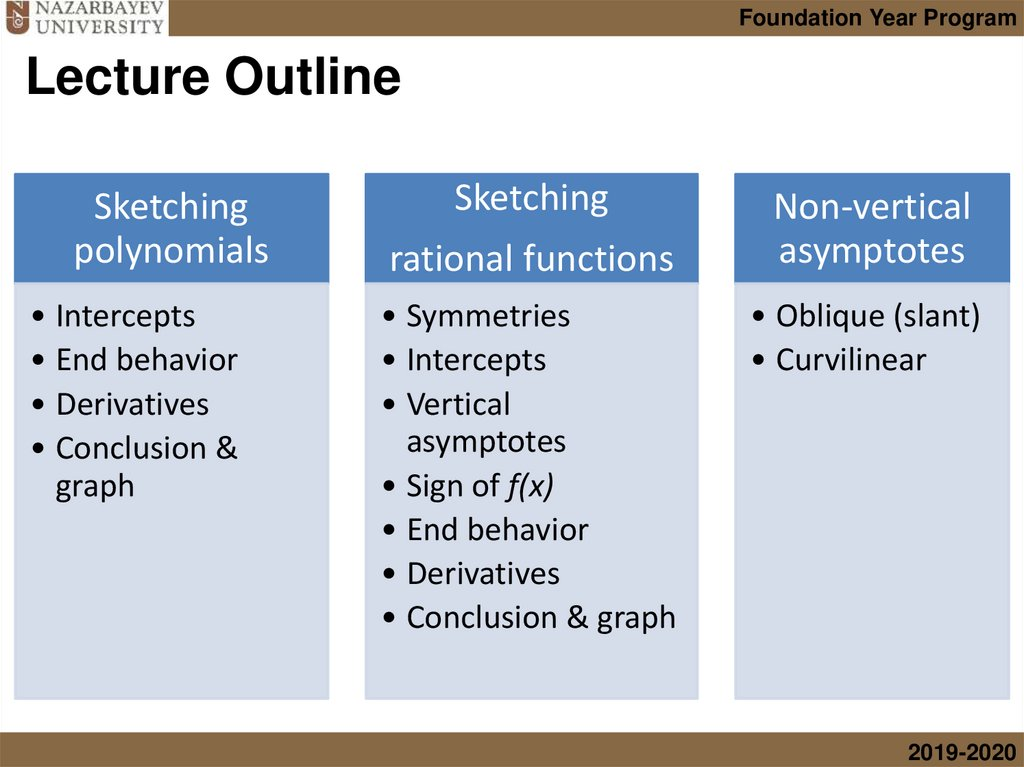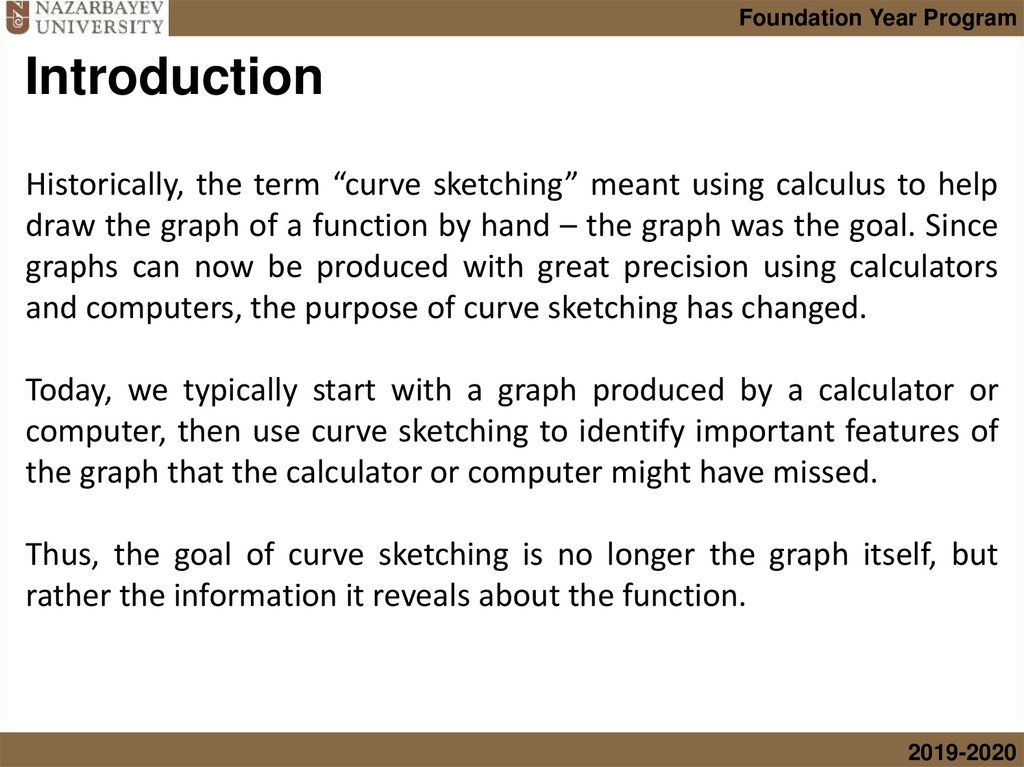# Differentiation. Sketching functions

## 1. 5.4 Differentiation 4. Sketching functions

Foundation Year Program
NUFYP Mathematics
5.4 Differentiation 4.
Sketching functions
Viktor Ten
2019-2020

## 2. Lecture Outline

Foundation Year Program
Lecture Outline
Sketching
polynomials
• Intercepts
• End behavior
• Derivatives
• Conclusion &
graph
Sketching
rational functions
• Symmetries
• Intercepts
• Vertical
asymptotes
• Sign of f(x)
• End behavior
• Derivatives
• Conclusion & graph
Non-vertical
asymptotes
• Oblique (slant)
• Curvilinear
2019-2020

## 3. Introduction

Foundation Year Program
Introduction
Historically, the term “curve sketching” meant using calculus to help
draw the graph of a function by hand – the graph was the goal. Since
graphs can now be produced with great precision using calculators
and computers, the purpose of curve sketching has changed.
Today, we typically start with a graph produced by a calculator or
computer, then use curve sketching to identify important features of
the graph that the calculator or computer might have missed.
Thus, the goal of curve sketching is no longer the graph itself, but
rather the information it reveals about the function.
2019-2020

## 4. Sketching polynomials

Foundation Year Program
Sketching polynomials
Define and describe (multiple or not) the roots of